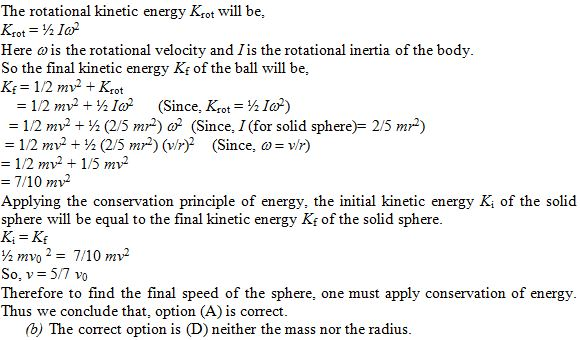×#### Thank you for registering.

One of our academic counsellors will contact you within 1 working day.

Click to Chat

1800-1023-196

+91-120-4616500

CART 0

• 0

MY CART (5)

Use Coupon: CART20 and get 20% off on all online Study Material

ITEM
DETAILS
MRP
DISCOUNT
FINAL PRICE
Total Price: Rs.

There are no items in this cart.
Continue Shopping
```
A solid sphere of mass m and radius r is projected horizontally out of a cannon without spinning with an initial speed v 0 . The sphere immediately lands on a level surface, where it skips a few times but eventually begins to roll without slipping. (a) To find the final speed of the sphere, one must apply (A) conservation of energy. (B) conservation of linear momentum. (C) conservation of angular momentum. (D) at least two of the previous principles. (b) The final speed for the sphere depends on (A) the radius. (B) the mass. (C) both the mass and the radius. (D) neither the mass nor the radius.
A solid sphere of mass m and radius r is projected horizontally out of a cannon without spinning with an initial speed v0. The sphere immediately lands on a level surface, where it skips a few times but eventually begins to roll without slipping.(a) To find the final speed of the sphere, one must apply(A) conservation of energy.(B) conservation of linear momentum.(C) conservation of angular momentum. (D) at least two of the previous principles.(b) The final speed for the sphere depends on(A) the radius. (B) the mass.(C) both the mass and the radius. (D) neither the mass nor the radius.

```
5 years ago

```							(a) The correct option is (A) conservation of energy.Let the initial kinetic Ki energy of the ball is, Ki = ½ mv0 2Here v0 is the initial velocity of the solid sphere and m is the mass of the solid sphere.After the sphere immediately lands on a level surface, where it eventually begins to roll without slipping, the final kinetic energy will be the sum of final linear kinetic energy (=1/2 mv2) having velocity v and rotational kinetic energy Krot.From the above observation, we noticed, the equation v = 5/7 v0 signifies that, the final speed of the solid sphere will only depend upon the initial speed of the sphere. Therefore the final speed for the sphere depends on neither the mass nor the radius. Thus option (D) is correct.
```
5 years ago
Think You Can Provide A Better Answer ?

## Other Related Questions on Mechanics

View all Questions »### Course Features

• 101 Video Lectures
• Revision Notes
• Previous Year Papers
• Mind Map
• Study Planner
• NCERT Solutions
• Discussion Forum
• Test paper with Video Solution### Course Features

• 110 Video Lectures
• Revision Notes
• Test paper with Video Solution
• Mind Map
• Study Planner
• NCERT Solutions
• Discussion Forum
• Previous Year Exam Questions